Home Practice
For learners and parents For teachers and schools
Textbooks
Full catalogue
Pricing SupportLog in

We think you are located in United States. Is this correct?

# 21.6 Description of motion

## 21.6 Description of motion (ESAHA)

The purpose of this chapter is to describe motion, and now that we understand the definitions of displacement, distance, velocity, speed and acceleration, we are ready to start using these ideas to describe how an object or person is moving. We will look at three ways of describing motion:

1. words

2. diagrams

3. graphs

These methods will be described in this section.

We will consider three types of motion: when the object is not moving (stationary object), when the object is moving at a constant velocity (uniform motion) and when the object is moving at a constant acceleration (motion at constant acceleration).

### Stationary Object (ESAHB)

The simplest motion that we can come across is that of a stationary object. A stationary object does not move and so its position does not change.

Consider an example, Vivian is waiting for a taxi. She is standing two metres from a stop street at $$t = \text{0}\text{ s}$$. After one minute, $$t = \text{60}\text{ s}$$, she is still $$\text{2}$$ metres from the stop street and after two minutes, at $$t = \text{120}\text{ s}$$, she is also $$\text{2}$$ metres from the stop street. Her position has not changed. Her displacement is zero (because his position is the same), her velocity is zero (because his displacement is zero) and her acceleration is also zero (because her velocity is not changing).

We can now draw graphs of position vs. time ($$\vec{x}$$ vs. $$t$$), velocity vs. time ($$\vec{v}$$ vs. $$t$$) and acceleration vs. time ($$\vec{a}$$ vs. $$t$$) for a stationary object. The graphs are shown below.Figure 21.2: Vivian stands at a stop sign.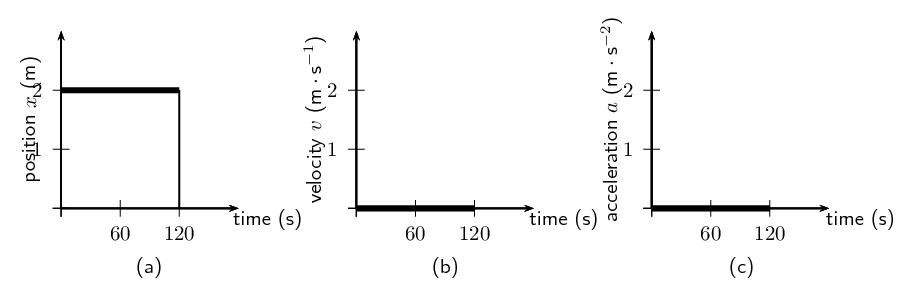Figure 21.3: Graphs for a stationary object (a) position vs. time (b) velocity vs. time (c) acceleration vs. time.

Vivian's position is $$\text{2}$$ metres in the positive direction from the stop street. If the stop street is taken as the reference point, her position remains at $$\text{2}$$ metres for $$\text{120}$$ seconds. The graph is a horizontal line at $$\text{2}$$ $$\text{m}$$. The velocity and acceleration graphs are also shown. They are both horizontal lines on the $$x$$-axis. Since her position is not changing, her velocity is $$\text{0}$$ $$\text{m·s^{-1}}$$ and since velocity is not changing, acceleration is $$\text{0}$$ $$\text{m·s^{-2}}$$.

(Recall from Mathematics) The gradient, $$m$$, of a line cna be calculated by dividing the change in the $$y$$-value (dependent variable) by the change in the $$x$$-value (independent variable). $$m=\frac{\Delta y}{\Delta x}$$

Since we know that velocity is the rate of change of position, we can confirm the value for the velocity vs. time graph, by calculating the gradient of the $$\vec{x}$$ vs. $$t$$ graph.

The gradient of a position vs. time graph gives the average velocity, while the tangent of a position vs. time graph gives the instantaneous velocity.

If we calculate the gradient of the $$\vec{x}$$ vs. $$t$$ graph for a stationary object we get:

\begin{align*} v & = \frac{\Delta \vec{x}}{\Delta t} \\ & = \frac{\vec{x}_{f} - \vec{x}_{i}}{t_{f} - t_{i}} \\ & = \frac{\text{2}\text{ m} - \text{2}\text{ m}}{\text{120}\text{ s} - \text{60}\text{ s}} \text{ (initial position } = \text{ final position)} \\ & = \text{0}\text{ m·s$^{-1}$} \text{ (for the time that Vivian is stationary)} \end{align*}

Similarly, we can confirm the value of the acceleration by calculating the gradient of the velocity vs. time graph.

The gradient of a velocity vs. time graph gives the average acceleration, while the tangent of a velocity vs. time graph gives the instantaneous acceleration.

If we calculate the gradient of the $$\vec{v}$$ vs. $$t$$ graph for a stationary object we get:

\begin{align*} a & = \frac{\Delta v}{\Delta t} \\ & = \frac{\vec{v}_{f} - \vec{v}_{i}}{t_{f} - t_{i}} \\ & = \frac{\text{0}\text{ m·s$^{-1}$} - \text{0}\text{ m·s$^{-1}$}}{\text{120}\text{ s} - \text{60}\text{ s}} \\ & = \text{0}\text{ m·s$^{-2}$} \end{align*}

Additionally, because the velocity vs. time graph is related to the position vs. time graph, we can use the area under the velocity vs. time graph to calculate the displacement of an object.

The area under the velocity vs. time graph gives the displacement.

The displacement of the object is given by the area under the graph, which is $$\text{0}$$ $$\text{m}$$. This is obvious, because the object is not moving.

### Motion at constant velocity (ESAHC)

Motion at a constant velocity or uniform motion means that the position of the object is changing at the same rate.

Assume that Vivian takes $$\text{100}$$ $$\text{s}$$ to walk the $$\text{100}$$ $$\text{m}$$ to the taxi-stop every morning. If we assume that Vivian's house is the origin and the direction to the taxi is positive, then Vivian's velocity is:

\begin{align*} v & = \frac{\Delta \vec{x}}{\Delta t} \\ & = \frac{{x}_{f} - {x}_{i}}{{t}_{f} - {t}_{i}} \\ & = \frac{\text{100}\text{ m} - \text{0}\text{ m}}{\text{100}\text{ s} - \text{0}\text{ s}} \\ & = \text{1}\text{ m·s$^{-1}$} \end{align*}

Vivian's velocity is $$\text{1}$$ $$\text{m·s^{-1}}$$. This means that she walked $$\text{1}$$ $$\text{m}$$ in the first second, another metre in the second second, and another in the third second, and so on. For example, after $$\text{50}$$ $$\text{s}$$ she will be $$\text{50}$$ $$\text{m}$$ from home. Her position increases by $$\text{1}$$ $$\text{m}$$ every $$\text{1}$$ $$\text{s}$$. A diagram of Vivian's position is shown below: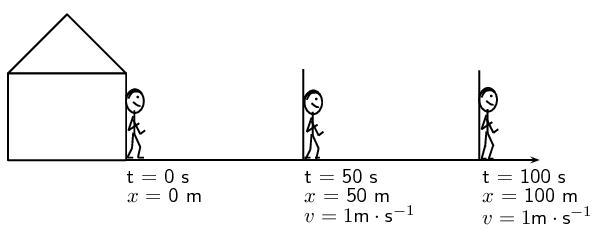We can now draw graphs of position vs.time ($$\vec{x}$$ vs. $$t$$), velocity vs. time ($$\vec{v}$$ vs. $$t$$) and acceleration vs. time ($$\vec{a}$$ vs. $$t$$) for Vivian moving at constant velocity. The graphs are shown here: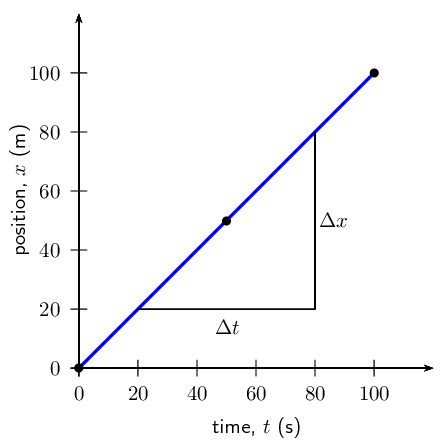Figure 21.4: Graphs for motion at constant velocity (a) position vs. time (b) velocity vs. time (c) acceleration vs. time. The area of the shaded portion in the $$v$$ vs. $$t$$ graph corresponds to the object's displacement.

In the evening Vivian walks $$\text{100}$$ $$\text{m}$$ from the bus stop to her house in $$\text{100}$$ $$\text{s}$$. Assume that Vivian's house is the origin. The following graphs can be drawn to describe the motion.Figure 21.5: Graphs for motion with a constant negative velocity. The area of the shaded portion in the $$v$$ vs. $$t$$ graph corresponds to the object's displacement.

We see that the $$\vec{v}$$ vs. $$t$$ graph is a horizontal line. If the velocity vs. time graph is a horizontal line, it means that the velocity is constant (not changing). Motion at a constant velocity is known as uniform motion. We can use the $$\vec{x}$$ vs. $$t$$ graph to calculate the velocity by finding the gradient of the line.

\begin{align*} v & = \frac{\Delta \vec{x}}{\Delta t} \\ & = \frac{{\vec{x}}_{f} - {\vec{x}}_{i}}{{t}_{f} - {t}_{i}} \\ & = \frac{\text{0}\text{ m} - \text{100}\text{ m}}{\text{100}\text{ s} - \text{0}\text{ s}} \\ & = -\text{1}\text{ m·s$^{-1}$} \end{align*}

Vivian has a velocity of $$-\text{1}$$ $$\text{m·s^{-1}}$$, or $$\text{1}$$ $$\text{m·s^{-1}}$$ towards her house. You will notice that the $$\vec{v}$$$$t$$$$-\text{1}$$ $$\text{m·s^{-1}}$$. The horizontal line means that the velocity stays the same (remains constant) during the motion. This is uniform velocity.

We can use the $$\vec{v}$$ vs. $$t$$ graph to calculate the acceleration by finding the gradient of the line.

\begin{align*} a & = \frac{\Delta \vec{v}}{\Delta t} \\ & = \frac{{\vec{v}}_{f} - {\vec{v}}_{i}}{{t}_{f} - {t}_{i}} \\ & = \frac{\text{1}\text{ m·s$^{-1}$} - \text{1}\text{ m·s$^{-1}$}}{\text{100}\text{ s} - \text{0}\text{ s}} \\ & = \text{0}\text{ m·s$^{-2}$} \end{align*}

Vivian has an acceleration of $$\text{0}$$ $$\text{m·s^{-2}}$$. You will notice that the graph of $$\vec{a}$$$$t$$$$\text{0}$$ $$\text{m·s^{-2}}$$. There is no acceleration during the motion because her velocity does not change.

We can use the $$\vec{v}$$ vs. $$t$$ graph to calculate the displacement by finding the area under the graph.

\begin{align*} \Delta \vec{x} & = \text{Area under graph} \\ & = l \times b \\ & = 100(-1) \\ & = -\text{100}\text{ m} \end{align*}

This means that Vivian has a displacement of $$\text{100}$$ $$\text{m}$$ towards her house.

## Velocity and acceleration

Textbook Exercise 21.5

Use the graphs in Figure 21.6 to calculate each of the following:

1. Calculate Vivian's velocity between $$\text{50}$$ $$\text{s}$$ and $$\text{100}$$ $$\text{s}$$ using the $$x$$ vs. $$t$$ graph. Hint: Find the gradient of the line.

2. Calculate Vivian's acceleration during the whole motion using the $$v$$ vs. $$t$$ graph.

3. Calculate Vivian's displacement during the whole motion using the $$v$$ vs. $$t$$ graph.

Solution not yet available

Thandi takes $$\text{200}$$ $$\text{s}$$ to walk $$\text{100}$$ $$\text{m}$$ to the bus stop every morning. In the evening Thandi takes $$\text{200}$$ $$\text{s}$$ to walk $$\text{100}$$ $$\text{m}$$ from the bus stop to her home.

1. Draw a graph of Thandi's position as a function of time for the morning (assuming that Thandi's home is the reference point). Use the gradient of the $$x$$ vs. $$t$$ graph to draw the graph of velocity vs. time. Use the gradient of the $$v$$ vs. $$t$$ graph to draw the graph of acceleration vs. time.

2. Draw a graph of Thandi's position as a function of time for the evening (assuming that Thandi's home is the origin). Use the gradient of the $$x$$ vs. $$t$$ graph to draw the graph of velocity vs. time. Use the gradient of the $$v$$ vs. $$t$$ graph to draw the graph of acceleration vs. time.

3. Discuss the differences between the two sets of graphs in questions 2 and 3.

Solution not yet available

## Motion at constant velocity

### Aim

To measure the position and time during motion at constant velocity and determine the average velocity as the gradient of a “Position vs. Time” graph.

### Apparatus

A battery operated toy car, stopwatch, meter stick or measuring tape.

### Method

1. Work with a friend. Copy the table below into your workbook.

2. Complete the table by timing the car as it travels each distance.

3. Time the car twice for each distance and take the average value as your accepted time.

4. Use the distance and average time values to plot a graph of “Distance vs. Time” onto graph paper. Stick the graph paper into your workbook. (Remember that “A vs. B” always means “$$y$$ vs. $$x$$”).

5. Insert all axis labels and units onto your graph.

6. Draw the best straight line through your data points.

7. Find the gradient of the straight line. This is the average velocity.

### Results

 Distance (m) Time (s) 1 2 Ave. $$\text{0}$$ $$\text{0,5}$$ $$\text{1,0}$$ $$\text{1,5}$$ $$\text{2,0}$$ $$\text{2,5}$$ $$\text{3,0}$$

### Conclusions

1. Did the car travel with a constant velocity?

2. How can you tell by looking at the “Distance vs. Time” graph if the velocity is constant?

3. How would the “Distance vs. Time” graph look for a car with a faster velocity?

4. How would the “Distance vs. Time” graph look for a car with a slower velocity?

### Motion at constant acceleration (ESAHD)

The final situation we will be studying is motion at constant acceleration. We know that acceleration is the rate of change of velocity. So, if we have a constant acceleration, this means that the velocity changes at a constant rate.

Let's look at our first example of Vivian waiting at the taxi stop again. A taxi arrived and Vivian got in. The taxi stopped at the stop street and then accelerated in the positive direction as follows: After $$\text{1}$$ $$\text{s}$$ the taxi covered a distance of $$\text{2,5}$$ $$\text{m}$$, after $$\text{2}$$ $$\text{s}$$ it covered $$\text{10}$$ $$\text{m}$$, after $$\text{3}$$ $$\text{s}$$ it covered $$\text{22,5}$$ $$\text{m}$$ and after $$\text{4}$$ $$\text{s}$$ it covered $$\text{40}$$ $$\text{m}$$. The taxi is covering a larger distance every second. This means that it is accelerating.

To calculate the velocity of the taxi you need to calculate the gradient of the line at each second:

\begin{align*} \vec{v}_{1s} & = \frac{\Delta \vec{x}}{\Delta t} \\ & = \frac{{\vec{x}}_{f} - {\vec{x}}_{i}}{{t}_{f} - {t}_{i}} \\ & = \frac{\text{5}\text{ m} - \text{0}\text{ m}}{\text{1,5}\text{ s} - \text{0,5}\text{ s}} \\ & = \text{5}\text{ m·s$^{-1}$} \end{align*}\begin{align*} \vec{v}_{2s} & = \frac{\Delta \vec{x}}{\Delta t} \\ & = \frac{{\vec{x}}_{f} - {\vec{x}}_{i}}{{t}_{f} - {t}_{i}} \\ & = \frac{\text{15}\text{ m} - \text{5}\text{ m}}{\text{2,5}\text{ s} - \text{1,5}\text{ s}} \\ & = \text{10}\text{ m·s$^{-1}$} \end{align*}\begin{align*} \vec{v}_{1s} & = \frac{\Delta \vec{x}}{\Delta t} \\ & = \frac{{\vec{x}}_{f} - {\vec{x}}_{i}}{{t}_{f} - {t}_{i}} \\ & = \frac{\text{30}\text{ m} - \text{15}\text{ m}}{\text{3,5}\text{ s} - \text{2,5}\text{ s}} \\ & = \text{15}\text{ m·s$^{-1}$} \end{align*}

From these velocities, we can draw the velocity-time graph which forms a straight line.

The acceleration is the gradient of the $$v$$ vs. $$t$$ graph and can be calculated as follows:

\begin{align*} a & = \frac{\Delta \vec{v}}{\Delta t} \\ & = \frac{{\vec{v}}_{f} - {\vec{v}}_{i}}{{t}_{f} - {t}_{i}} \\ & = \frac{\text{15}\text{ m·s$^{-1}$} - \text{5}\text{ m·s$^{-1}$}}{\text{3}\text{ s} - \text{1}\text{ s}} \\ & = \text{5}\text{ m·s$^{-2}$} \end{align*}

The acceleration does not change during the motion (the gradient stays constant). This is motion at constant or uniform acceleration.

The graphs for this situation are shown below: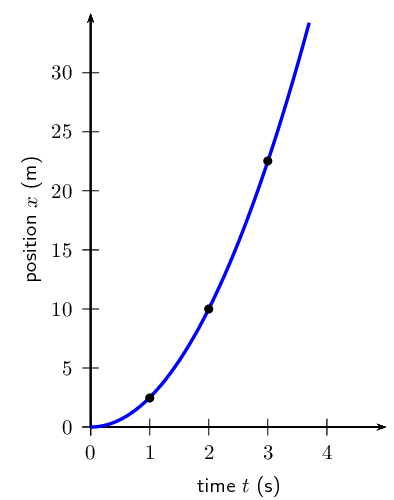Figure 21.6: Graphs for motion with a constant acceleration starting from rest.

#### Velocity from acceleration vs. time graphs

Just as we used velocity vs. time graphs to find displacement, we can use acceleration vs. time graphs to find the velocity of an object at a given moment in time. We simply calculate the area under the acceleration vs. time graph, at a given time. In the graph below, showing an object at a constant positive acceleration, the increase in velocity of the object after $$\text{2}$$ seconds corresponds to the shaded portion.

\begin{align*} v = \text{ area of rectangle } & = a\times \Delta t \\ & = \text{5}\text{ m·s$^{-2}$} \times \text{2}\text{ s} \\ & = \text{10}\text{ m·s$^{-1}$} \end{align*}

The velocity of the object at $$t = \text{2}\text{ s}$$ is therefore $$\text{10}$$ $$\text{m·s^{-1}}$$.

### Summary of graphs (ESAHE)

The relation between graphs of position, velocity and acceleration as functions of time is summarised in the next figure.

You will also often be required to draw graphs based on a description of the motion in words or from a diagram. Remember that these are just different methods of presenting the same information. If you keep in mind the general shapes of the graphs for the different types of motion, there should not be any difficulty with explaining what is happening.

The description of the motion represented by a graph should include the following (where possible):

1. whether the object is moving in the positive or negative direction

2. whether the object is at rest, moving at constant velocity or moving at constant positive acceleration (speeding up) or constant negative acceleration (slowing down)

## Position versus time using a ticker timer

### Aim

To measure the position and time during motion and to use that data to plot a “Position vs. Time” graph.

### Apparatus

Trolley, ticker tape apparatus, tape, graph paper, ruler, ramp

### Method

1. Work with a friend. Copy the table below into your workbook.

2. Attach a length of tape to the trolley.

3. Run the other end of the tape through the ticker timer.

4. Start the ticker timer going and roll the trolley down the ramp.

5. Repeat steps 1–3.

6. On each piece of tape, measure the distance between successive dots. Note these distances in the table below.

7. Use the frequency of the ticker timer to work out the time intervals between successive dots. Note these times in the table below,

8. Work out the average values for distance and time.

9. Use the average distance and average time values to plot a graph of “Distance vs. Time” onto graph paper. Stick the graph paper into your workbook. (Remember that “A vs. B” always means “$$y$$ vs. $$x$$”).

10. Insert all axis labels and units onto your graph.

11. Draw the best straight line through your data points.

### Results

 Distance (m) Time (s) 1 2 Ave. 1 2 Ave.

### Discussion

Describe the motion of the trolley down the ramp.

### Worked examples (ESAHF)

The worked examples in this section demonstrate the types of questions that can be asked about graphs.

## Worked example 3: Description of motion based on a position-time graph

The position vs. time graph for the motion of a car is given below. Draw the corresponding velocity vs. time and acceleration vs. time graphs, and then describe the motion of the car.### Identify what information is given and what is asked for

The question gives a position vs. time graph and the following three things are required:

1. Draw a $$v$$ vs. $$t$$ graph.

2. Draw an $$a$$ vs. $$t$$ graph.

3. Describe the motion of the car.

To answer these questions, break the motion up into three sections: 0 – $$\text{2}$$ seconds, $$\text{2}$$ – $$\text{4}$$ seconds and $$\text{4}$$ – $$\text{6}$$ seconds.

### Velocity vs. time graph for $$\text{0}$$ – $$\text{2}$$ seconds

For the first $$\text{2}$$ seconds we can see that the position (and hence the displacement) remains constant - so the object is not moving, thus it has zero velocity during this time. We can reach this conclusion by another path too: remember that the gradient of a displacement vs. time graph is the velocity. For the first $$\text{2}$$ seconds we can see that the displacement vs. time graph is a horizontal line, i.e.. it has a gradient of zero. Thus the velocity during this time is zero and the object is stationary.

### Velocity vs. time graph for $$\text{2}$$ – $$\text{4}$$ seconds

For the next $$\text{2}$$ seconds, displacement is increasing with time so the object is moving. Looking at the gradient of the displacement graph we can see that it is not constant. In fact, the slope is getting steeper (the gradient is increasing) as time goes on. Thus, remembering that the gradient of a displacement vs. time graph is the velocity, the velocity must be increasing with time during this phase.

### Velocity vs. time graph for $$\text{4}$$ – $$\text{6}$$ seconds

For the final $$\text{2}$$ seconds we see that displacement is still increasing with time, but this time the gradient is constant, so we know that the object is now travelling at a constant velocity, thus the velocity vs. time graph will be a horizontal line during this stage. We can now draw the graphs:

So our velocity vs. time graph looks like this one below. Because we haven't been given any values on the vertical axis of the displacement vs. time graph, we cannot figure out what the exact gradients are and therefore what the values of the velocities are. In this type of question it is just important to show whether velocities are positive or negative, increasing, decreasing or constant.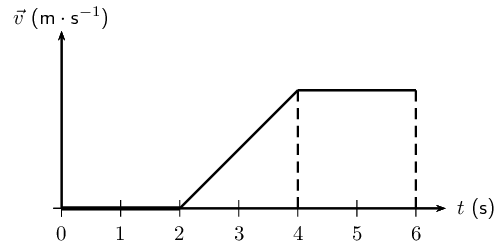Once we have the velocity vs. time graph its much easier to get the acceleration vs. time graph as we know that the gradient of a velocity vs. time graph is the just the acceleration.

### Acceleration vs. time graph for 0 – $$\text{2}$$ seconds

For the first $$\text{2}$$ seconds the velocity vs. time graph is horizontal and has a value of zero, thus it has a gradient of zero and there is no acceleration during this time. (This makes sense because we know from the displacement time graph that the object is stationary during this time, so it can't be accelerating).

### Acceleration vs. time graph for $$\text{2}$$ – $$\text{4}$$ seconds

For the next $$\text{2}$$ seconds the velocity vs. time graph has a positive gradient. This gradient is not changing (i.e. its constant) throughout these $$\text{2}$$ seconds so there must be a constant positive acceleration.

### Acceleration vs. time graph for $$\text{4}$$ – $$\text{6}$$ seconds

For the final $$\text{2}$$ seconds the object is travelling with a constant velocity. During this time the gradient of the velocity vs. time graph is once again zero, and thus the object is not accelerating. The acceleration vs. time graph looks like this:### A description of the object's motion

A brief description of the motion of the object could read something like this: At $$t = \text{0}\text{ s}$$ the object is stationary at some position and remains stationary until $$t = \text{2}\text{ s}$$ when it begins accelerating. It accelerates in a positive direction for $$\text{2}$$ $$\text{s}$$ until $$t = \text{4}\text{ s}$$ and then travels at a constant velocity for a further $$\text{2}$$ $$\text{s}$$.

## Worked example 4: Calculations from a velocity vs. time graph

The velocity vs. time graph of a truck is plotted below. Calculate the distance and displacement of the truck after $$\text{15}$$ seconds.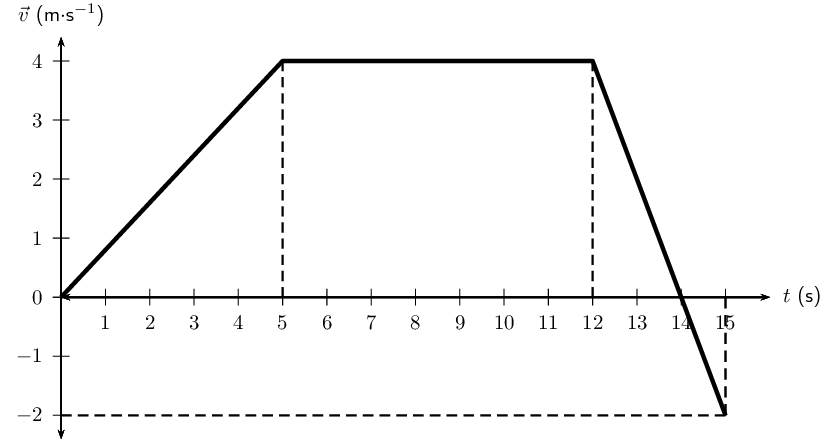### Decide how to tackle the problem

We are asked to calculate the distance and displacement of the car. All we need to remember here is that we can use the area between the velocity vs. time graph and the time axis to determine the distance and displacement.

### Determine the area under the velocity vs. time graph

Break the motion up: $$\text{0}$$ – $$\text{5}$$ seconds, $$\text{5}$$ – $$\text{12}$$ seconds, $$\text{12}$$ – $$\text{14}$$ seconds and $$\text{14}$$ – $$\text{15}$$ seconds.

For $$\text{0}$$ – $$\text{5}$$ seconds: The displacement is equal to the area of the triangle on the left:

\begin{align*} {\text{Area}}_{△} & = \frac{1}{2}b\times h \\ & = \frac{1}{2}\times \text{5}\text{ s} \times \text{4}\text{ m·s$^{-1}$} \\ & = \text{10}\text{ m} \end{align*}

For $$\text{5}$$ – $$\text{12}$$ seconds: The displacement is equal to the area of the rectangle:

\begin{align*} {\text{Area}}_{\square } & = l \times b \\ & = \text{7}\text{ s} \times \text{4}\text{ m·s$^{-1}$} \\ & = \text{28}\text{ m} \end{align*}

For $$\text{12}$$ – $$\text{14}$$ seconds the displacement is equal to the area of the triangle above the time axis on the right:

\begin{align*} {\text{Area}}_{△} & = \frac{1}{2}b\times h \\ & = \frac{1}{2}\times \text{2}\text{ s} \times \text{4}\text{ m·s$^{-1}$} \\ & = \text{4}\text{ m} \end{align*}

For $$\text{14}$$ – $$\text{15}$$ seconds the displacement is equal to the area of the triangle below the time axis:

\begin{align*} {\text{Area}}_{△} & = \frac{1}{2}b\times h \\ & = \frac{1}{2}\times \text{1}\text{ s} \times \text{2}\text{ m·s$^{-1}$} \\ & = \text{1}\text{ m} \end{align*}

### Determine the total distance of the car

Now the total distance of the car is the sum of all of these areas:

\begin{align*} D & = \text{10}\text{ m} + \text{28}\text{ m} + \text{4}\text{ m} + \text{1}\text{ m} \\ & = \text{43}\text{ m} \end{align*}

### Determine the total displacement of the car

Now the total displacement of the car is just the sum of all of these areas. HOWEVER, because in the last second (from $$t = \text{14}\text{ s}$$ to $$t = \text{15}\text{ s}$$) the velocity of the car is negative, it means that the car was going in the opposite direction, i.e. back where it came from! So, to find the total displacement, we have to add the first $$\text{3}$$ areas (those with positive displacements) and subtract the last one (because it is a displacement in the opposite direction).

\begin{align*} \Delta \vec{x} & = \text{10}\text{ m} + \text{28}\text{ m} + \text{4}\text{ m} - \text{1}\text{ m} \\ & = \text{41}\text{ m} \text{ in the positive direction} \end{align*}

## Worked example 5: Velocity from a position vs. time graph

The position vs. time graph below describes the motion of an athlete.1. What is the velocity of the athlete during the first $$\text{4}$$ seconds?

2. What is the velocity of the athlete from $$t = \text{4}\text{ s}$$ to $$t = \text{7}\text{ s}$$?

### The velocity during the first $$\text{4}$$ seconds

The velocity is given by the gradient of a position vs. time graph. During the first $$\text{4}$$ seconds, this is

\begin{align*} \vec{v} & = \frac{\Delta \vec{x}}{\Delta t} \\ & = \frac{\text{4}\text{ m} - \text{0}\text{ m}}{\text{4}\text{ s} - \text{0}\text{ s}} \\ & = \text{1}\text{ m·s$^{-1}$} \end{align*}

### The velocity during the last 3 seconds

For the last 3 seconds we can see that the displacement stays constant. The graph shows a horizontal line and therefore the gradient is zero. Thus $$v = \text{0}\text{ m·s^{-1}}$$.

## Worked example 6: Drawing a $$v$$ vs. $$t$$ graph from an $$a$$ vs. $$t$$ graph

The acceleration vs. time graph for a car starting from rest, is given below. Calculate the velocity of the car and hence draw the velocity vs. time graph.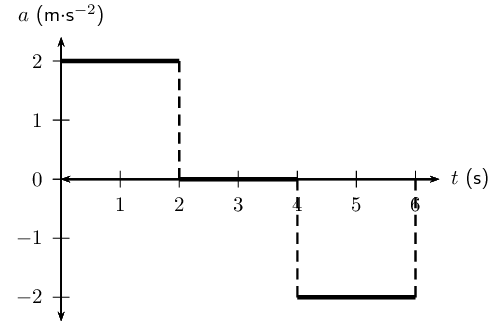### Calculate the change in velocity values by using the area under each part of the graph.

The motion of the car can be divided into three time sections: $$\text{0}$$ – $$\text{2}$$ seconds; $$\text{2}$$ – $$\text{4}$$ seconds and $$\text{4}$$ – $$\text{6}$$ seconds. To be able to draw the velocity vs. time graph, the change in velocity for each time section needs to be calculated. The change in velocity is equal to the area of the square under the graph:

For $$\text{0}$$ – $$\text{2}$$ seconds:

\begin{align*} \Delta v = {\text{Area}}_{\square } & = l \times b \\ & = \text{2}\text{ s} \times \text{2}\text{ m·s$^{-2}$} \\ & = \text{4}\text{ m·s$^{-1}$} \end{align*}

The change in velocity of the car from $$t = 0 \text{ s}$$ to $$t = 2 \text{ s}$$ is $$\text{4}$$ $$\text{m·s^{-1}}$$.

For $$\text{2}$$ – $$\text{4}$$ seconds:

\begin{align*} \Delta v = {\text{Area}}_{\square } & = l \times b \\ & = \text{2}\text{ s} \times \text{0}\text{ m·s$^{-2}$} \\ & = \text{0}\text{ m·s$^{-1}$} \end{align*}

The change in velocity is $$\text{0}$$ $$\text{m·s^{-1}}$$ from $$t = \text{2}\text{ s}$$ to $$t = \text{4}\text{ s}$$. In other words, the velocity remains constant during this time interval.

For $$\text{4}$$ – $$\text{6}$$ seconds:

\begin{align*} \Delta v = {\text{Area}}_{\square } & = l \times b \\ & = \text{2}\text{ s} \times -\text{2}\text{ m·s$^{-2}$} \\ & = -\text{4}\text{ m·s$^{-1}$} \end{align*}

The acceleration had a negative value, which means that the velocity decreased. It started with a velocity of $$\text{4}$$ $$\text{m·s^{-1}}$$ at $$t = 4 \text{ s}$$ and the velocity decreased by $$4 \text{ m·s^{-1}}$$ to give a final velocity of $$0 \text{ m·s^{-1}}$$ at $$t = 6 \text{ s}$$.

### Now use the values to draw the velocity vs. time graph.

The velocity vs. time graph looks like this: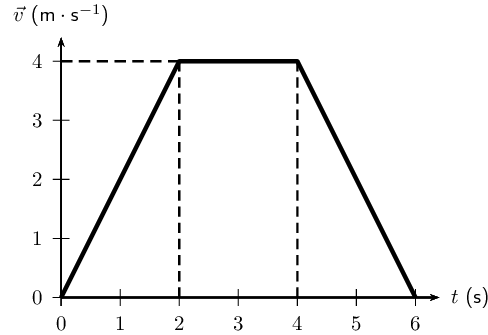## Graphs

Textbook Exercise 21.6

A car is parked $$\text{10}$$ $$\text{m}$$ from home for $$\text{10}$$ minutes. Draw a displacement-time, velocity-time and acceleration-time graphs for the motion. Label all the axes.

Solution not yet available

A bus travels at a constant velocity of $$\text{12}$$ $$\text{m·s^{-1}}$$ for $$\text{6}$$ seconds. Draw the displacement-time, velocity-time and acceleration-time graph for the motion. Label all the axes.

Solution not yet available

An athlete runs with a constant acceleration of $$\text{1}$$ $$\text{m·s^{-2}}$$ for $$\text{4}$$ $$\text{s}$$. Draw the acceleration-time, velocity-time and displacement time graphs for the motion. Accurate values are only needed for the acceleration-time and velocity-time graphs.

Solution not yet available

The following velocity-time graph describes the motion of a car. Draw the displacement-time graph and the acceleration-time graph and explain the motion of the car according to the three graphs.

Solution not yet available

The following velocity-time graph describes the motion of a truck. Draw the displacement-time graph and the acceleration-time graph and explain the motion of the truck according to the three graphs.

Solution not yet available